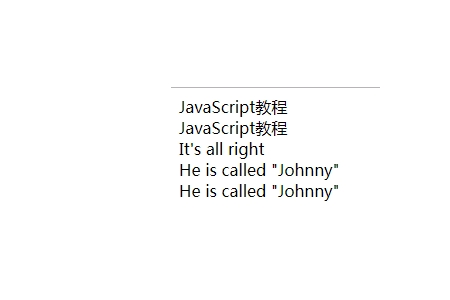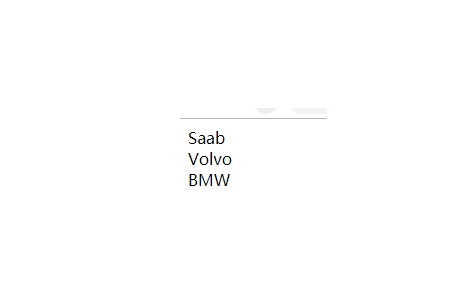# JavaScript 数据类型JavaScript的数据类型有：字符串、数字、布尔、数组、对象、Null、Undefined前端控 更新时间：2019-02-12 22:02:02

JavaScript 数据类型

JavaScript 拥有动态类型

JavaScript 拥有动态类型。这意味着相同的变量可用作不同的类型：

```var x; // x 为 undefined
var x = 6; // x 为数字
var x = "Bill"; // x 为字符串```

JavaScript 字符串

```var coursename="JavaScript教程";
var coursename='JavaScript教程';```

## 实例

```<script>
var coursename1="JavaScript教程";
var coursename2='JavaScript教程';
document.write(coursename1 + "<br>")
document.write(coursename2 + "<br>")
</script>```JavaScript 数字

JavaScript 只有一种数字类型。数字可以带小数点，也可以不带：

```var x1=34.00;//使用小数点来写
var x2=34; //不使用小数点来写```

```var y=123e5;      // 12300000
var z=123e-5;     // 0.00123```

JavaScript 布尔

```var x=true;
var y=false;```

JavaScript 数组

```var cars=new Array();
cars="Saab";
cars="Volvo";
cars="BMW";```

`var cars=new Array("Saab","Volvo","BMW");`

## 实例

```<script>
var i;
var cars = new Array();
cars = "Saab";
cars = "Volvo";
cars = "BMW";

for (i=0;i<cars.length;i++)
{
document.write(cars[i] + "<br>");
}
</script>```JavaScript 对象

`var person={firstname:"John", lastname:"Doe", id:5566};`

```var person={
firstname  : "John",
lastname  : "Doe",
id       ： 5566
};```

## 实例

```<script>
var person=
{
firstname : "John",
lastname  : "Doe",
id        :  5566
};
document.write(person.firstname + "<br>");
document.write(person["firstname"] + "<br>");
</script>```Undefined 和 Null

Undefined 这个值表示变量不含有值。

```cars=null;
person=null;```

```var carname=new String;
var x=new Number;
var y=new Boolean;
var cars=new Array;
var person= new Object;```

php中文网
phpstudy

QQ交流群